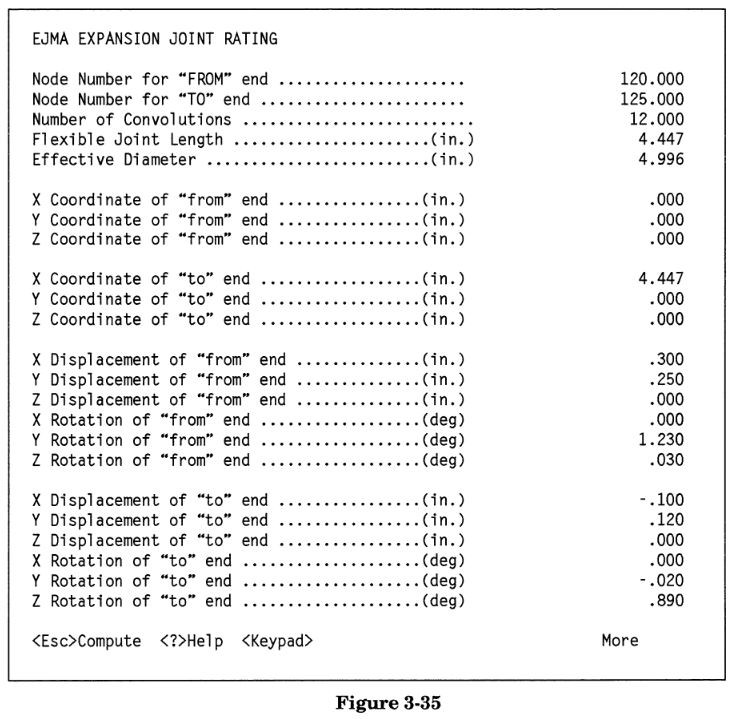Search

# 3.3.3 Use of the ERATE Program CAESAR II

For more complex configurations, the relative expansion joint end displacements can be evaluated using an auxiliary routine (accessible from the WRC 297/SIF/Flange option of CAESAR II). Full instructions for the use of this routine are found in the CAESAR II User's Manual.

This routine requires 23 data items (with a single value per line), describing the orientation, length, and end displacements of the expansion joint; from this, it calculates the relative displacements. The required items are described below:The input for the current example is shown in Figure 3-35.Output for this problem is shown in Figure 3-36.Results — e(total) — are given in terms of equivalent axial displacement per convolution — in this case, 0.083 inches per convolution. The accuracy of the initial estimate (done without the benefit of the ERATE program) of an equivalent axial extension of 0.904 inches can be determined by multiplying 0.083 times 12 convolutions, for a total equivalent extension of 0.996 inches (an error of approximately 9%). It can be seen that the bulk of the error is due to the underestimation of the lateral displacement (see x, y, theta, tors — the total axial, lateral, rotational, and torsional displacements respectively on the bellows as a whole) on the expansion joint — the estimate was 0.13 inches, while ERATE calculated it as 0.158 inches. This is important, since lateral loading is very critical. Additionally, without the use of a calculational aid such as ERATE, the lateral displacement will be the most difficult to estimate, especially as the rotational angle increases.

Torsion on the expansion joint should be approximately zero. If not, it is recommended that the acceptability of the bellows for the torsional load be verified by contacting the expansion joint manufacturer.

Modeling And Analysis Of The Piping System

Located in Calgary Alberta Canada; We serve our clients across USA and Canada.